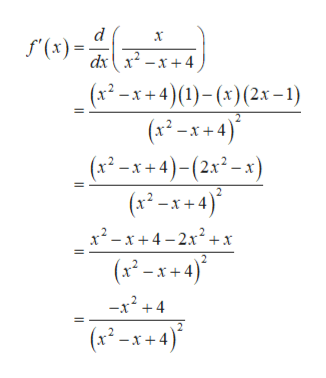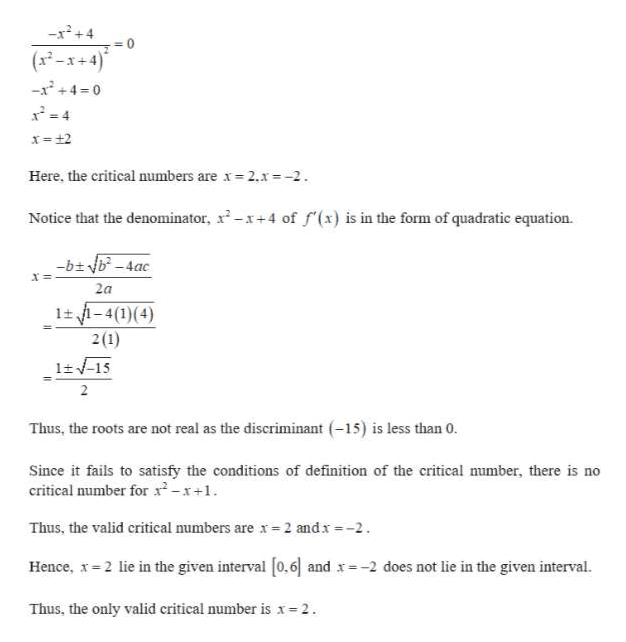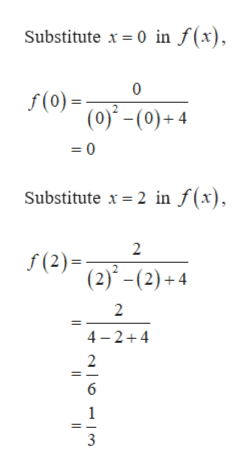# Find the absolute maximum and absolute minimum values of f on the given interval.f(x) =x/(x2-x+4),  [0, 6]

Question
11 views

Find the absolute maximum and absolute minimum values of f on the given interval.

f(x) =x/(x2-x+4),  [0, 6]
check_circle

Step 1

Obtain the first derivative of the given function.help_outlineImage Transcriptionclosed f(x) dx2-x+4 (x2-x+4)(1)-(x)(2x -1) (x2-x+4) (x-x+4)-(2x -x) (x x+4)* 2 x2 -x+4-2x2+x (x-x+4)* 2 -x2+4 (xx+4) Il fullscreen
Step 2

Set f ′(x) = 0 and obtain the critical numbers.help_outlineImage Transcriptionclose-x2+4 0 (x2-x+4) -x2+4=0 x 2 Here, the critical numbers are x 2.x -2 Notice that the denominator, 2-x+4 of f (x) is in the form of quadratic equation. -bb-4ac 2a 1 -4(1)(4) 2(1) 115 2 Thus, the roots are not real as the discriminant (15) is less than 0. Since it fails to satisfy the conditions of definition of the critical mumber, there is no critical number for -x+1 Thus, the valid critical numbers are x 2 andx-2 Hence, x 2 lie in the given interval [0.6 and x-2 does not lie in the given interval. Thus, the only valid critical number is x-2 fullscreen
Step 3

Apply the extreme values of the given interval and the ...help_outlineImage TranscriptioncloseSubstitute x 0 in f(x) 0 f(0) (0)-(0)+4 =0 Substitute x 2 in f(x) 2 f(2) (2)-(2)+4 2 4-2+4 2 6 fullscreen

### Want to see the full answer?

See Solution

#### Want to see this answer and more?

Solutions are written by subject experts who are available 24/7. Questions are typically answered within 1 hour.*

See Solution
*Response times may vary by subject and question.
Tagged in

### Other